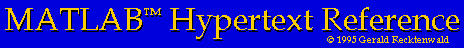# Matrices

Matrices are the fundamental data type in MATLAB.

## Creating matrices

To create a matrix you simply introduce it on the left hand side of an equal sign. Of course this means that the expression on the right side of the equal sign must evaluate to a matrix.

For example the statment

```	>> A = [1 2; 3 4]
```
creates the 2 by 2 matrix A
```	A =
1  2
3  4
```

## Addressing matrix elements

Matrix elements are referred to with subscript notation. If A is a matrix, then A(2,3) is the element in the second row and third column.

The following statements create a 3 by 3 matrix, print the (2,3) element and change the (3,2) element.

```	>> A = [1 2 3; 4 5 6; 7 8 9]
A =
1   2   3
4   5   6
7   8   9

>> A(2,3)             %  ask MATLAB to print the (2,3) element
ans =
6

>> A(3,2) = -5         %  reassign the (2,3) element
A =
1   2   3
4   5   6
7  -5   9

```

## Matrix operations

• Addition and subtraction
• Operations with scalars
• Operations with vectors
• Matrix Multiplication
• Element-wise matrix operations
• Passing matrices to built-in functions